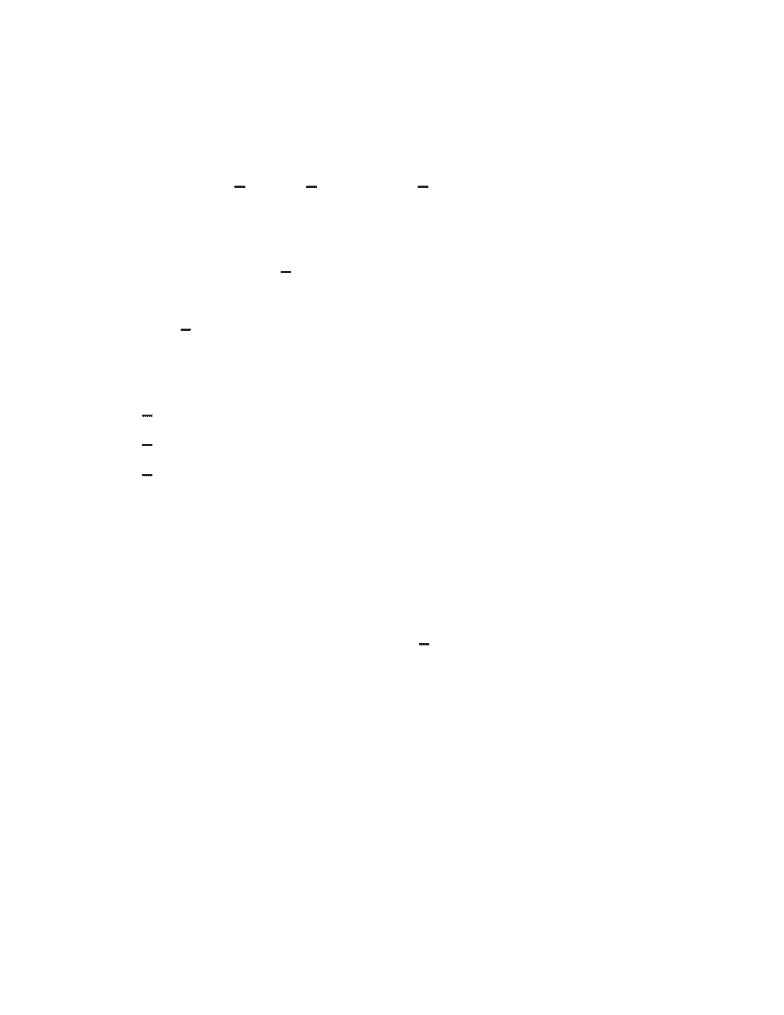Study Guides (390,000)
US (220,000)
UC-Irvine (3,000)
MATH (600)
MATH 2B (100)
Midterm

# MATH 2B Study Guide - Midterm Guide: Blood Type, Intermediate Value Theorem, Exponential FunctionPremium

Department
Mathematics
Course Code
MATH 2B
Professor
Timmy Ma
Study Guide
Midterm

This preview shows pages 1-3. to view the full 47 pages of the document.
University of California
Irvine
MATH 2B
Single-Variable Calculus
Fall 2017
Term Test 1
Prof: Timmy Ma
Exam Guide

Only pages 1-3 are available for preview. Some parts have been intentionally blurred.

Subscribers OnlyFrom Math 2A, we know how to take the derivative of a function. For example,
x x , (x) 2x ,
d
dx
2= 2 d
dx
2+ 1 = (x) 2x
d
dx
2+ π =
In general, for any constant C,
(x) 2x
d
dx
2+c=
We can view , as an operator on the space of functions.
d
dx
Derivatives:
x x
d
dx
2= 2
(x) 2x
d
dx
2+ 1 =
(x) 2x
d
dx
2+c=
Antiderivative:
- A function or functions F(x) such that F’(x) = 2x
In general, the antiderivative of a function is given by F(x) + c, where F(x) is one of the
antiderivatives of a given f(x). That is F’(x) = f(x) or F(x) = f(x)
d
dx
Example
1:
Find the most general antiderivatives of each of the following functions.
a. f(x) = sinx
b. f(x) = 1/x
c. f(x) = x4  , n -1=/
Antiderivatives Review

Only pages 1-3 are available for preview. Some parts have been intentionally blurred.

Subscribers OnlySolution:
a. F(x) = -cosx is an antiderivative of f(x) = sinx. Thus, the most general antiderivative is G(x)
= -cosx + c.
b. Recall that lnx = 1/x, but this is only valid when x > 0. If  x =/= 0, then we have
d
dx
d
dx
ln|x| = 1/x. Thus the most general antiderivatives of 1/x is G(x) = ln|x|+c
c. We note that . Thus the most general antiderivative is G(x) = (x) x
d
dx
1
n+1 n+1 = 4x
1
n+1 n+1
+c
*Need to be familiar with the antiderivative table on page 352.
____________________________________________________________________________
Example
2:
Find all functions g such that g’(x) = 4 sinx + x
2x
5x
Solution:
We first rewrite the function as g’(x) = 4sinx + 2x4 - 1
x
Thus the antiderivative is g(x) = -4cosx + x
5
25− 2x+c
____________________________________________________________________________
Example
3:
Find f if and f(0) = -2(x) 0(1 )f=ex+ 2 + x2−1
Solution:
(x) 0f=ex+ 2 1
1+x2
Thus: (x) e0tan xf = x+ 2 −1 +c
Since f(0) = -2, we have 0tan 0 −> c 2 = e0+ 2 −1 +c = 3
Thus: (x) e0tan xf = x+ 2 −1 − 3
____________________________________________________________________________